Like   Tweet   +1
 /* styles */

## September 2018

 table div table+table+table+table div table{width:100%;padding:0}table div table+table+table+table div table img{width:96.23%;padding:0;float:none}table div table+table+table+table div table td{width:100%;padding:0 1.88% 18px}/* styles */## REMC1 Resource Request Form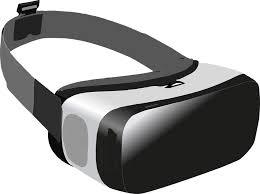Did you know REMC1 has several technology resources that you can check out and use in your classroom? The items include virtual reality devices, classroom maker tools, starlab and much more! Available items can now be viewed and requested by completing the Resource Request Form (also linked on the REMC1 webpage).

 table div table+table+table+table+table+table+table div table{width:100%;padding:0}table div table+table+table+table+table+table+table div table img{width:96.23%;padding:0;float:none}table div table+table+table+table+table+table+table div table td{width:100%;padding:0 1.88% 18px}/* styles */## Upcoming Training Workshops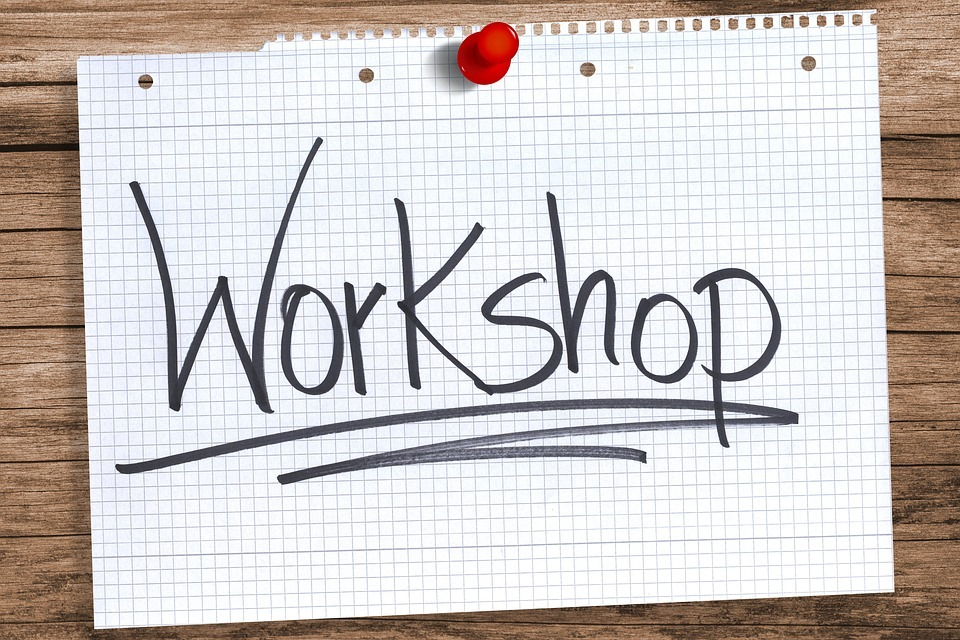Please join us for one of our many upcoming workshops that will teach you about new technology resources that can be implemented in your classroom. The full listing of events offered this fall can be viewed at www.remc1.org/professional-development. A few of the workshops we want to highlight are as follows:

UP Classroom Makers Workshop
CCISD: October 16
9 a.m. - 3 p.m.

Virtual Reality in the Classroom
GOISD: October 5
CCISD: November 2
9:00 a.m. - 3:00 p.m.

CCISD: November 6
GOISD: November 7
8:30 a.m. - 11:45 a.m.

Digital Age Teaching and Learning
CCISD: November 6
GOISD: November 7
12:15 p.m. - 3:30 p.m.

 table div table+table+table+table+table+table+table+table+table+table div table{width:100%;padding:0}table div table+table+table+table+table+table+table+table+table+table div table img{width:96.23%;padding:0;float:none}table div table+table+table+table+table+table+table+table+table+table div table td{width:100%;padding:0 1.88% 18px}/* styles */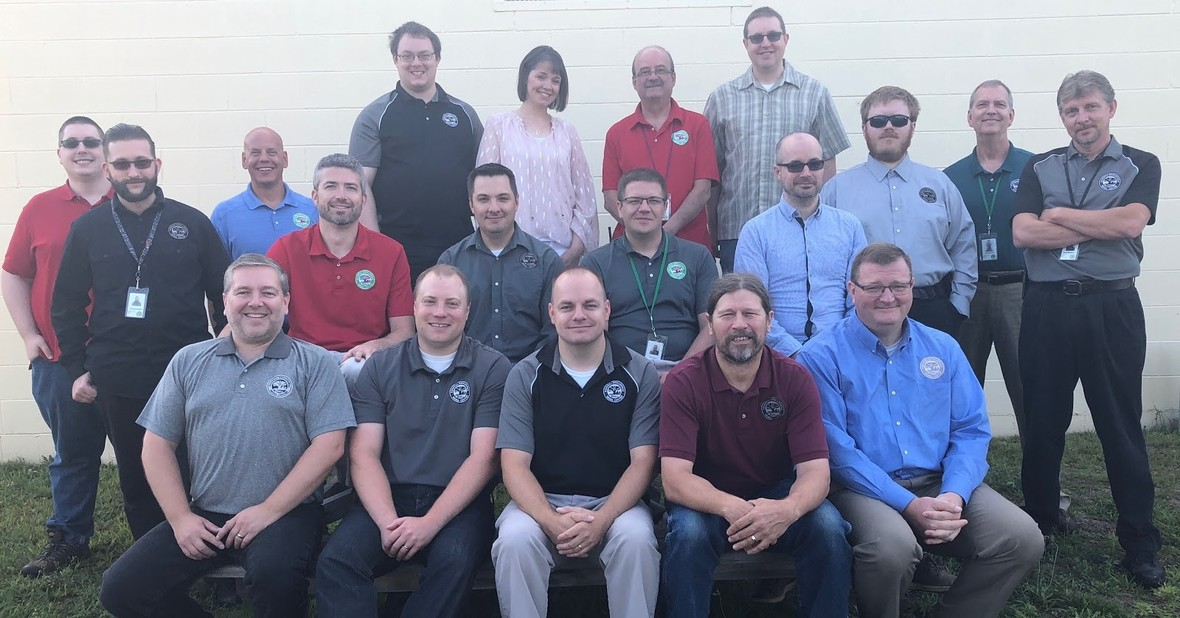We are proud supporters of the Copper Country and Gogebic-Ontonagon ISDs and happy to serve our local school districts and community, and the entire Western UP.

 table div table+table+table+table+table+table+table+table+table+table+table+table+table div table{width:100%;padding:0}table div table+table+table+table+table+table+table+table+table+table+table+table+table div table img{width:96.23%;padding:0;float:none}table div table+table+table+table+table+table+table+table+table+table+table+table+table div table td{width:100%;padding:0 1.88% 18px}/* styles */## State Tech StandardsMDE has replaced the old METS tech standards with updated Student Technology Competencies, MiTECs.

The REMC Association has created MiTechKids to help with meeting those new competencies in K-5 , as well as continuing to update the national standards-based 21Things4Students.

 table div table+table+table+table+table+table+table+table+table+table+table+table+table+table+table+table div table{width:100%;padding:0}table div table+table+table+table+table+table+table+table+table+table+table+table+table+table+table+table div table img{width:96.23%;padding:0;float:none}table div table+table+table+table+table+table+table+table+table+table+table+table+table+table+table+table div table td{width:100%;padding:0 1.88% 18px}/* styles */## Discovery Streaming (on demand videos for your classroom)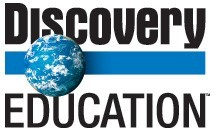Every REMC member has access to Discovery Education (formerly United Streaming) at http://www.discoveryeducation.com/

This valuable resource gives educators access to Digital Textbooks, Streaming Media, Professional Development and a vast online community.

If you do not have a login please submit a ticket to REMC1 Helpdesk and we will get you setup!

Individual student accounts can also be created, and we can assist with that.

 table div table+table+table+table+table+table+table+table+table+table+table+table+table+table+table+table+table+table+table div table{width:100%;padding:0}table div table+table+table+table+table+table+table+table+table+table+table+table+table+table+table+table+table+table+table div table img{width:96.23%;padding:0;float:none}table div table+table+table+table+table+table+table+table+table+table+table+table+table+table+table+table+table+table+table div table td{width:100%;padding:0 1.88% 18px}/* styles */## Staff Spotlight!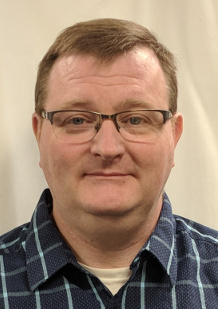This month we are spotlighting Tom Griffin who began working at REMC1 in April. Tom graduated from MTU with a Bachelor's Degree in Mechanical Engineering. After graduation, he worked at MTU as a System Administrator for the School of Business and Economics as well as Educational Opportunity. He then transitioned to Computer Mechanix in 2003 where he worked until joining our team. Welcome Tom!

 table div table+table+table+table+table+table+table+table+table+table+table+table+table+table+table+table+table+table+table+table+table+table div table{width:100%;padding:0}table div table+table+table+table+table+table+table+table+table+table+table+table+table+table+table+table+table+table+table+table+table+table div table img{width:96.23%;padding:0;float:none}table div table+table+table+table+table+table+table+table+table+table+table+table+table+table+table+table+table+table+table+table+table+table div table td{width:100%;padding:0 1.88% 18px}/* styles */## SupportNet Helpdesk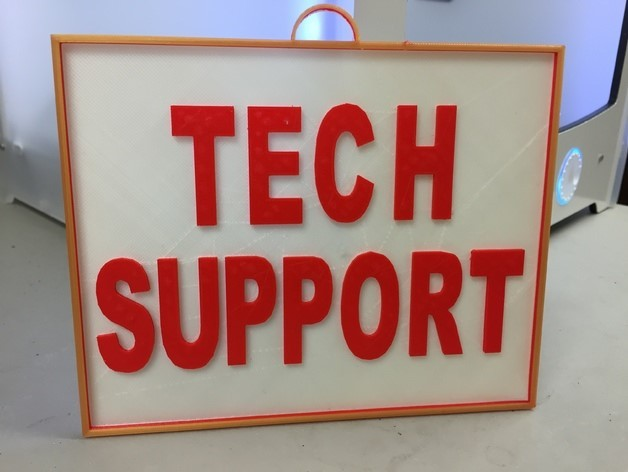We have multiple methods which you can use to contact support and receive assistance. Please follow this link which describes the various ways you can receive timely support: https://confluence.remc1.net/display/PS/How+to+use+Supportnet

Each district computer should also have a red Support tag identifying the computer number, that also has our phone number, email and web addresses for easy reference.

Each completed support ticket email has a link to a satisfaction survey. We take this feedback seriously, and appreciate your time completing them.

The REMC1 Supportnet Team

Onsite support team schedule

Helpdesk schedule

 table div table+table+table+table+table+table+table+table+table+table+table+table+table+table+table+table+table+table+table+table+table+table+table+table+table div table{width:100%;padding:0}table div table+table+table+table+table+table+table+table+table+table+table+table+table+table+table+table+table+table+table+table+table+table+table+table+table div table img{width:96.23%;padding:0;float:none}table div table+table+table+table+table+table+table+table+table+table+table+table+table+table+table+table+table+table+table+table+table+table+table+table+table div table td{width:100%;padding:0 1.88% 18px}/* styles */## Teacher's CornerREMC1 Resources
Poster printer - up to 36” wide banners can be printed and sent to your district, even with options for weather resistant outdoor paper.

Starlab - an inflatable planetarium trained staff can use in your district, allowing students to crawl inside and enjoy a wide assortment of experiences.

Virtual Reality kit - trained staff can check out the VR kit and take students on virtual expeditions all over the world and beyond.

Maker kit - an assortment of coding, robotics, and makerspace resources are available for checkout.

Cutout maker - an electronic ‘die cut’ tool with thousands of shapes for creating bulletin boards, manipulatives, and a variety of displays. This can be accessed from your own computer, and cutouts in any size and color can be delivered to your district
https://www.variquest.com/product/cutout-maker/

 table div table+table+table+table+table+table+table+table+table+table+table+table+table+table+table+table+table+table+table+table+table+table+table+table+table+table+table+table div table{width:100%;padding:0}table div table+table+table+table+table+table+table+table+table+table+table+table+table+table+table+table+table+table+table+table+table+table+table+table+table+table+table+table div table img{width:96.23%;padding:0;float:none}table div table+table+table+table+table+table+table+table+table+table+table+table+table+table+table+table+table+table+table+table+table+table+table+table+table+table+table+table div table td{width:100%;padding:0 1.88% 18px}/* styles */## Resources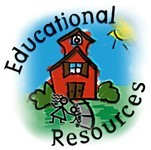EduPaths is a free resource from TRIG created to centralize PD around the state. Find resources and get FREE SCECH hours!

Current REMC Connect (REMC Association of Michigan Newsletter)

Check out Discovery Streaming updates and enhancing instruction with streaming resources.

MeL is a great place for Michigan Teachers to find classroom resources. They have just recently launched MeL Teachers which offers eight centers that will help with site navigation and make important information easier to access.

REMC Virtual Courses, October, 2018. Free to Michigan school personnel. Sessions include: Open Education Resources, Google Apps for Chrome, Literacy and Technology, Social Media and more. SCECHs available. FAQs about the free REMC Virtual Courses

eSchool News-Teaching and Learning

 table div table+table+table+table+table+table+table+table+table+table+table+table+table+table+table+table+table+table+table+table+table+table+table+table+table+table+table+table+table+table+table div table{width:100%;padding:0}table div table+table+table+table+table+table+table+table+table+table+table+table+table+table+table+table+table+table+table+table+table+table+table+table+table+table+table+table+table+table+table div table img{width:96.23%;padding:0;float:none}table div table+table+table+table+table+table+table+table+table+table+table+table+table+table+table+table+table+table+table+table+table+table+table+table+table+table+table+table+table+table+table div table td{width:100%;padding:0 1.88% 18px}/* styles */## Connection CornerDiscovery Education upcoming events
Video Conference field trips and Classroom collaborations
iNACOL webinars
ISTE blog and magazine
EdTech magazine
REMC SAVE
Michigan Ed Tech - METS

 table div table+table+table+table+table+table+table+table+table+table+table+table+table+table+table+table+table+table+table+table+table+table+table+table+table+table+table+table+table+table+table+table+table+table div table{width:100%;padding:0}table div table+table+table+table+table+table+table+table+table+table+table+table+table+table+table+table+table+table+table+table+table+table+table+table+table+table+table+table+table+table+table+table+table+table div table img{width:96.23%;padding:0;float:none}table div table+table+table+table+table+table+table+table+table+table+table+table+table+table+table+table+table+table+table+table+table+table+table+table+table+table+table+table+table+table+table+table+table+table div table td{width:100%;padding:0 1.88% 18px}/* styles */table div table+table+table+table+table+table+table+table+table+table+table+table+table+table+table+table+table+table+table+table+table+table+table+table+table+table+table+table+table+table+table+table+table+table+table+table div table{width:100%;padding:0}table div table+table+table+table+table+table+table+table+table+table+table+table+table+table+table+table+table+table+table+table+table+table+table+table+table+table+table+table+table+table+table+table+table+table+table+table div table img{width:96.23%;padding:0;float:none}table div table+table+table+table+table+table+table+table+table+table+table+table+table+table+table+table+table+table+table+table+table+table+table+table+table+table+table+table+table+table+table+table+table+table+table+table div table td{width:100%;padding:0 1.88% 18px}/* styles */table div table+table+table+table+table+table+table+table+table+table+table+table+table+table+table+table+table+table+table+table+table+table+table+table+table+table+table+table+table+table+table+table+table+table+table+table+table+table div table{width:100%;padding:0}table div table+table+table+table+table+table+table+table+table+table+table+table+table+table+table+table+table+table+table+table+table+table+table+table+table+table+table+table+table+table+table+table+table+table+table+table+table+table div table img{width:96.23%;padding:0;float:none}table div table+table+table+table+table+table+table+table+table+table+table+table+table+table+table+table+table+table+table+table+table+table+table+table+table+table+table+table+table+table+table+table+table+table+table+table+table+table div table td{width:100%;padding:0 1.88% 18px}/* styles */table div table+table+table+table+table+table+table+table+table+table+table+table+table+table+table+table+table+table+table+table+table+table+table+table+table+table+table+table+table+table+table+table+table+table+table+table+table+table+table+table div table{width:100%;padding:0}table div table+table+table+table+table+table+table+table+table+table+table+table+table+table+table+table+table+table+table+table+table+table+table+table+table+table+table+table+table+table+table+table+table+table+table+table+table+table+table+table div table img{width:96.23%;padding:0;float:none}table div table+table+table+table+table+table+table+table+table+table+table+table+table+table+table+table+table+table+table+table+table+table+table+table+table+table+table+table+table+table+table+table+table+table+table+table+table+table+table+table div table td{width:100%;padding:0 1.88% 18px}/* styles */